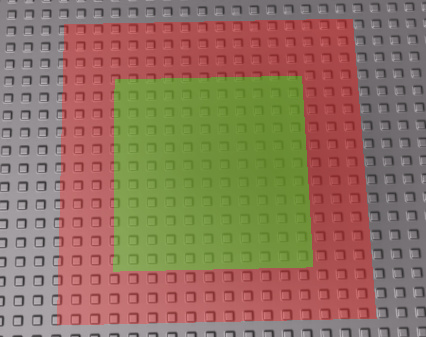# Check if a player is in range of a part by at least 70%

Using magnituide, how can I determine if a player is at least 70% in range based on the part’s area / size?The Red Represents the part’s size, the Green is the 70% mark that I wish to detect using magnitude.
Basically, how can I use magnitude to do an if statement to detemine if the player is 70% inside that part.

Also a problem is I might not be able to use Magnitude, as that is a Circle.

if (char.HumanoidRootPart.Position - part.position).magnitude < [70% statement math] then
end

2 Likes

if (char.HumanoidRootPart.Position - Part.Position).magnitude < (Part.Size.X*0.7) then

This will only work properly if the part is square.
Also it will do it in a circular area from the centre of the part. You’ll need to do a lot more math to figure out if it’s inside the square area or use a different API.

1 Like

Yeah I’m trying to see if there is a way for more complex shapes

Sorry for the misleading picture (it’s a square in the pic)

I’ll use Region3 to solve this problem.

1 Like

If the part is a square and is not rotated then you can use the `isClose` method:

``````char.HumanoidRootPart.Position:isClose(part.Position, part.Size.X/2*.7)
``````
2 Likes

If the part needs to rotate then Region3 is insufficient. I suggest making an invisible collider part.

2 Likes

Not necessary, fairly redundant to be exact. He could simply use some maths to tell if objects are colliding.

1 Like

I’ve done the math before and you just end up writing a rectangular prisim collision algorithm which already exists by adding a collider part. It’s even more redundant to use math, and much slower.

1 Like

What you could try is getting the top right and bottom left corners of the shape and use their Y and Z coordinates, and then track the player’s position until their HumanoidRootPart’s position is within the two coordinates. Obviously this would be super laggy for if there’s a lot of parts in the game using this method, but with only one shouldn’t be too bad.

1 Like
``````local relativePos = part.CFrame:pointToObjectSpace(humanoidRootPart.Position)
if math.abs(relativePos.X) <= part.Size.X * 0.7 * 0.5 and math.abs(relativePos.Z) <= part.Size.Z * 0.7 * 0.5 then
print("inside")
end
``````

This will correctly handle rotated parts. You can also check the Y axis in exactly the same way if you want 3D checking, but your original question kind of implied you didn’t.

24 Likes

Don’t even need to do that, it’s basic CFrame math

1 Like

If it’s so basic, why didn’t you post an example?

12 Likes

i am interested in your method as i do not know of an easier OBB vs Point test

1 Like

Yeah, was my fault for even posting here. Not the one to share code all the time.

1 Like